The OpenFOAM FoundationperfectFluid< Specie > Class Template Reference

Perfect gas equation of state. More...

Inheritance diagram for perfectFluid< Specie >: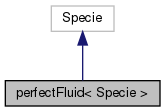[legend]
Collaboration diagram for perfectFluid< Specie >:[legend]

## Public Member Functions

perfectFluid (const Specie &sp, const scalar R, const scalar rho0)
Construct from components. More...

perfectFluid (const dictionary &dict)
Construct from dictionary. More...

perfectFluid (const word &name, const perfectFluid &)
Construct as named copy. More...

autoPtr< perfectFluidclone () const
Construct and return a clone. More...

scalar R () const
Return fluid constant [J/kg/K]. More...

scalar rho (scalar p, scalar T) const
Return density [kg/m^3]. More...

scalar H (const scalar p, const scalar T) const
Return enthalpy departure [J/kg]. More...

scalar Cp (scalar p, scalar T) const
Return Cp departure [J/(kg K]. More...

scalar E (const scalar p, const scalar T) const
Return internal energy departure [J/kg]. More...

scalar Cv (scalar p, scalar T) const
Return Cv departure [J/(kg K]. More...

scalar S (const scalar p, const scalar T) const
Return entropy [J/kg/K]. More...

scalar psi (scalar p, scalar T) const
Return compressibility rho/p [s^2/m^2]. More...

scalar Z (scalar p, scalar T) const
Return compression factor []. More...

scalar CpMCv (scalar p, scalar T) const
Return (Cp - Cv) [J/(kg K]. More...

void write (Ostream &os) const
Write to Ostream. More...

void operator+= (const perfectFluid &)

void operator*= (const scalar)

## Static Public Member Functions

static autoPtr< perfectFluidNew (const dictionary &dict)

static word typeName ()
Return the instantiated type name. More...

## Static Public Attributes

static const bool incompressible = false
Is the equation of state is incompressible i.e. rho != f(p) More...

static const bool isochoric = false
Is the equation of state is isochoric i.e. rho = const. More...

## Friends

perfectFluid operator+ (const perfectFluid &, const perfectFluid &)

perfectFluid operator* (const scalar s, const perfectFluid &)

perfectFluid operator== (const perfectFluid &, const perfectFluid &)

Ostreamoperator (Ostream &, const perfectFluid &)

## Detailed Description

### template<class Specie> class Foam::perfectFluid< Specie >

Perfect gas equation of state.

Source files

Definition at line 47 of file perfectFluid.H.

## ◆ perfectFluid() [1/3]

 perfectFluid ( const Specie & sp, const scalar R, const scalar rho0 )
inline

Construct from components.

Definition at line 32 of file perfectFluidI.H.

References Foam::name().

Here is the call graph for this function: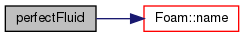## ◆ perfectFluid() [2/3]

 perfectFluid ( const dictionary & dict )

Construct from dictionary.

Definition at line 32 of file perfectFluid.C.

## ◆ perfectFluid() [3/3]

 perfectFluid ( const word & name, const perfectFluid< Specie > & )
inline

Construct as named copy.

## ◆ clone()

 Foam::autoPtr< Foam::perfectFluid< Specie > > clone ( ) const
inline

Construct and return a clone.

Definition at line 61 of file perfectFluidI.H.

References perfectFluid< Specie >::New().

Here is the call graph for this function: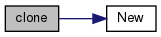## ◆ New()

 Foam::autoPtr< Foam::perfectFluid< Specie > > New ( const dictionary & dict )
inlinestatic

Definition at line 70 of file perfectFluidI.H.

References dict.

Referenced by perfectFluid< Specie >::clone().

Here is the caller graph for this function: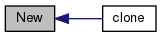## ◆ typeName()

 static word typeName ( )
inlinestatic

Return the instantiated type name.

Definition at line 124 of file perfectFluid.H.

## ◆ R()

 Foam::scalar R ( ) const
inline

Return fluid constant [J/kg/K].

Definition at line 81 of file perfectFluidI.H.

## ◆ rho()

 Foam::scalar rho ( scalar p, scalar T ) const
inline

Return density [kg/m^3].

Definition at line 88 of file perfectFluidI.H.

References R, and T.

## ◆ H()

 Foam::scalar H ( const scalar p, const scalar T ) const
inline

Return enthalpy departure [J/kg].

Definition at line 95 of file perfectFluidI.H.

References Foam::constant::standard::Pstd, and rho.

## ◆ Cp()

 Foam::scalar Cp ( scalar p, scalar T ) const
inline

Return Cp departure [J/(kg K].

Definition at line 102 of file perfectFluidI.H.

References Foam::constant::standard::Pstd, R, rho, and Foam::sqr().

Here is the call graph for this function: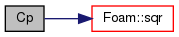## ◆ E()

 Foam::scalar E ( const scalar p, const scalar T ) const
inline

Return internal energy departure [J/kg].

Definition at line 111 of file perfectFluidI.H.

## ◆ Cv()

 Foam::scalar Cv ( scalar p, scalar T ) const
inline

Return Cv departure [J/(kg K].

Definition at line 118 of file perfectFluidI.H.

## ◆ S()

 Foam::scalar S ( const scalar p, const scalar T ) const
inline

Return entropy [J/kg/K].

Definition at line 125 of file perfectFluidI.H.

References Foam::log(), Foam::constant::standard::Pstd, and R.

Here is the call graph for this function: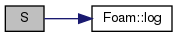## ◆ psi()

 Foam::scalar psi ( scalar p, scalar T ) const
inline

Return compressibility rho/p [s^2/m^2].

Definition at line 132 of file perfectFluidI.H.

References R, and T.

## ◆ Z()

 Foam::scalar Z ( scalar p, scalar T ) const
inline

Return compression factor [].

Definition at line 139 of file perfectFluidI.H.

## ◆ CpMCv()

 Foam::scalar CpMCv ( scalar p, scalar T ) const
inline

Return (Cp - Cv) [J/(kg K].

Definition at line 146 of file perfectFluidI.H.

References R, rho, and Foam::sqr().

Here is the call graph for this function: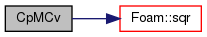## ◆ write()

 void write ( Ostream & os ) const

Write to Ostream.

Definition at line 43 of file perfectFluid.C.

Here is the call graph for this function: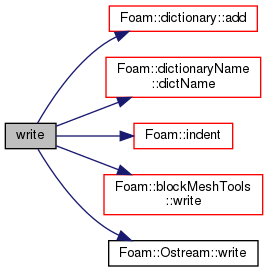## ◆ operator+=()

 void operator+= ( const perfectFluid< Specie > & )
inline

Definition at line 159 of file perfectFluidI.H.

References Foam::mag(), and Y.

Here is the call graph for this function: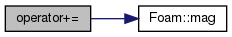## ◆ operator*=()

 void operator*= ( const scalar s )
inline

Definition at line 178 of file perfectFluidI.H.

References Foam::mag(), and s().

Here is the call graph for this function: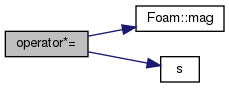## ◆ operator+

 perfectFluid operator+ ( const perfectFluid< Specie > & , const perfectFluid< Specie > & )
friend

## ◆ operator*

 perfectFluid operator* ( const scalar s, const perfectFluid< Specie > & )
friend

## ◆ operator==

 perfectFluid operator== ( const perfectFluid< Specie > & , const perfectFluid< Specie > & )
friend

## ◆ operator

 Ostream& operator ( Ostream & , const perfectFluid< Specie > & )
friend

## ◆ incompressible

 const bool incompressible = false
static

Is the equation of state is incompressible i.e. rho != f(p)

Definition at line 133 of file perfectFluid.H.

## ◆ isochoric

 const bool isochoric = false
static

Is the equation of state is isochoric i.e. rho = const.

Definition at line 136 of file perfectFluid.H.

The documentation for this class was generated from the following files:
• src/thermophysicalModels/specie/equationOfState/perfectFluid/perfectFluid.H
• src/thermophysicalModels/specie/equationOfState/perfectFluid/perfectFluid.C
• src/thermophysicalModels/specie/equationOfState/perfectFluid/perfectFluidI.H